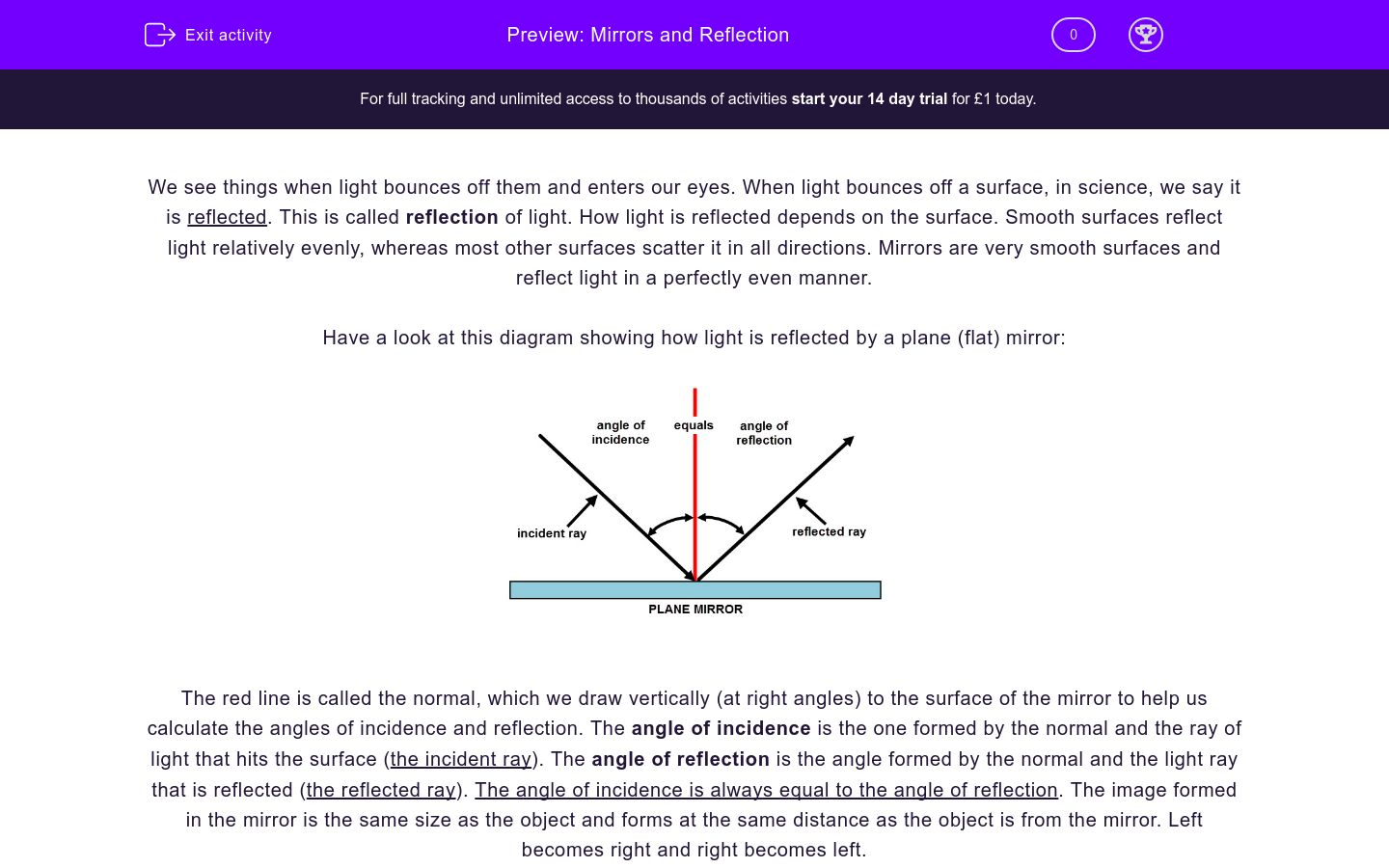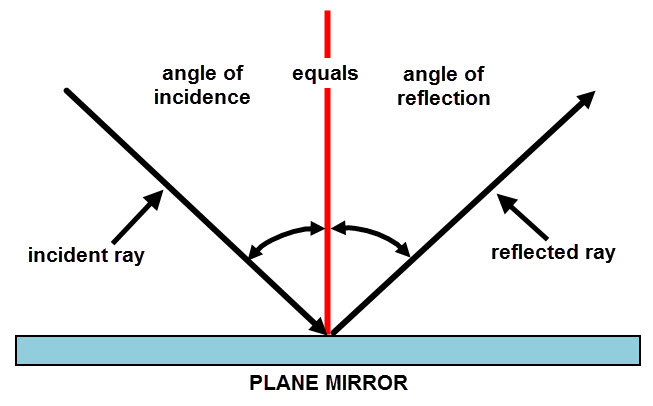# Mirrors and Reflection

In this worksheet, students will explore how mirrors work and the reflection of light by different surfaces.Key stage:  KS 3

Curriculum topic:   Physics: Waves

Curriculum subtopic:   Light Waves

Difficulty level:### QUESTION 1 of 10

We see things when light bounces off them and enters our eyes. When light bounces off a surface, in science, we say it is reflected. This is called reflection of light. How light is reflected depends on the surface. Smooth surfaces reflect light relatively evenly, whereas most other surfaces scatter it in all directions. Mirrors are very smooth surfaces and reflect light in a perfectly even manner.

Have a look at this diagram showing how light is reflected by a plane (flat) mirror:The red line is called the normal, which we draw vertically (at right angles) to the surface of the mirror to help us calculate the angles of incidence and reflection. The angle of incidence is the one formed by the normal and the ray of light that hits the surface (the incident ray). The angle of reflection is the angle formed by the normal and the light ray that is reflected (the reflected ray). The angle of incidence is always equal to the angle of reflection. The image formed in the mirror is the same size as the object and forms at the same distance as the object is from the mirror. Left becomes right and right becomes left.

A kaleidoscope is a toy used to make lots of reflections of something. When you look into it, it can look a little like this:What is the scientific word for light bouncing off a surface?

refraction

reflection

dispersion

Is the following statement true or false?

A smooth surface reflects light to different directions.

true

false

Is the following statement true or false?

A plane mirror is not smooth.

true

false

Fill in the missing words in the sentence below by ticking one option from the list below:

The image formed in a mirror is the _________ size and the ___________ distance as the object.

bigger - same

smaller - longer

same - same

Is the following statement true or false?

true

false

Complete the following sentence by choosing one of the options below:

A kaleidoscope is a toy that........

uses mirrors to create lots of images of something.

uses mirrors to scatter light to different directions.

uses light to make things appear brighter.

Is the following statement true or false?

The incident ray is bigger than the reflected ray.

true

false

Complete the sentence by selecting one of the options below:

The angle of incidence is always ________ to the angle of reflection.

smaller

equal

bigger

Why do we use a normal line?

To help us view the light rays.

To help us measure the angles of incidence and reflection.

To help us see a mirror image.

Fill in the gaps in the following sentence by selecting one of the options from the list below:

The angle of incidence is the angle between the _________ and the ____________ ray.

normal - incident

normal - reflected

incident - reflected

• Question 1

What is the scientific word for light bouncing off a surface?

reflection
EDDIE SAYS
Light bounces off surfaces and this is called reflection.
• Question 2

Is the following statement true or false?

A smooth surface reflects light to different directions.

false
EDDIE SAYS
Smooth surfaces reflect light evenly.
• Question 3

Is the following statement true or false?

A plane mirror is not smooth.

false
EDDIE SAYS
Plane mirrors are very smooth and reflect light perfectly.
• Question 4

Fill in the missing words in the sentence below by ticking one option from the list below:

The image formed in a mirror is the _________ size and the ___________ distance as the object.

same - same
EDDIE SAYS
Images formed in mirrors are the same size and the same distance as the object.
• Question 5

Is the following statement true or false?

false
EDDIE SAYS
In a mirror left becomes right and right becomes left.
• Question 6

Complete the following sentence by choosing one of the options below:

A kaleidoscope is a toy that........

uses mirrors to create lots of images of something.
EDDIE SAYS
A kaleidoscope uses mirrors to create lots of images of something.
• Question 7

Is the following statement true or false?

The incident ray is bigger than the reflected ray.

false
EDDIE SAYS
The incident ray and the reflected ray always have the same angle, either side of the normal line. Imagine kicking a football at an angle against a smooth wall - where will it bounce off to? It will bounce off at the same angle (the other side of 'normal') as that which it hit the wall.
• Question 8

Complete the sentence by selecting one of the options below:

The angle of incidence is always ________ to the angle of reflection.

equal
EDDIE SAYS
The angle of incidence is always equal to the angle of reflection.
• Question 9

Why do we use a normal line?

To help us measure the angles of incidence and reflection.
EDDIE SAYS
The normal is drawn to help us measure the angles of incidence and reflection.
• Question 10

Fill in the gaps in the following sentence by selecting one of the options from the list below:

The angle of incidence is the angle between the _________ and the ____________ ray.

normal - incident
EDDIE SAYS
The angle of incidence is the angle formed between the normal and the incident ray.
---- OR ----

Sign up for a £1 trial so you can track and measure your child's progress on this activity.

### What is EdPlace?

We're your National Curriculum aligned online education content provider helping each child succeed in English, maths and science from year 1 to GCSE. With an EdPlace account you’ll be able to track and measure progress, helping each child achieve their best. We build confidence and attainment by personalising each child’s learning at a level that suits them.

Get started